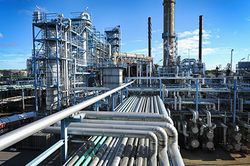# Documentation:CHBE Exam Wiki/Midterm Exam 2 2016W/MT2 Question 2

CHBE 241
Exam resources wikiChemical and Biological Engineering
Welcome to the CHBE Exam Resources Wiki!

This wiki is intended to host past exams

with fully worked-out hints and solutions
Past Exams
Final Exam 2016W
Midterm Exam 1 2016W
Midterm Exam 2 2016W
Problem Sets
Module 1 - Process Basics
Module 2 - Reactors
Module 3 - Separations 1
Module 4 - Separations 2
Module 5 - Non-reactive Energy Balances
Module 6 - Reactive Energy Balances

Oil extracted from a well may contain a significant amount of water. This water is typically removed in a 3 phase separator which splits the entering stream into 2 liquid streams and a gas stream. To simplify this system, we will assume the entering stream contains only water (H2O), n-butane (C4H10) and n-octane (C8H18) and that the streams exit the separator at equilibrium.Total pressure in the separator is 6.382 bar. You may also assume that water and octane are not miscible. Make sure to list any other assumptions that you make.
The following information is given:

• Unit operates at 60°C
• MWwater = 18 g/mol ; MWbutane = 58 g/mol ; MWoctane = 114 g/mol
• p*water = 149.61 mmHg ; p*butane = 6.2 bar
• Hbutane(at 60°C) = 0.000403 molbutane/(kgwater·bar)
• Kc(mass fractions at 60°C) = ${\frac {(w_{butane})_{octanephase}}{(w_{butane})_{waterphase}}}$The Antoine equation is: $log_{10}p^{*}(bar)=A-{\frac {B}{T(K)+C}}$Table 1: Antoine equation coefficients for octane, respectively

Compound A B C
Octane 4.049 1355 -63.63

## Question 2a [5 points]

What is the vapour pressure of octane?

## Question 2b [10 points]

What is mass fraction of all components in the liquid water phase?

## Question 2c [5 points]

What is the mass fraction of all components the octane rich liquid?

Script error: The function "navbox" does not exist.